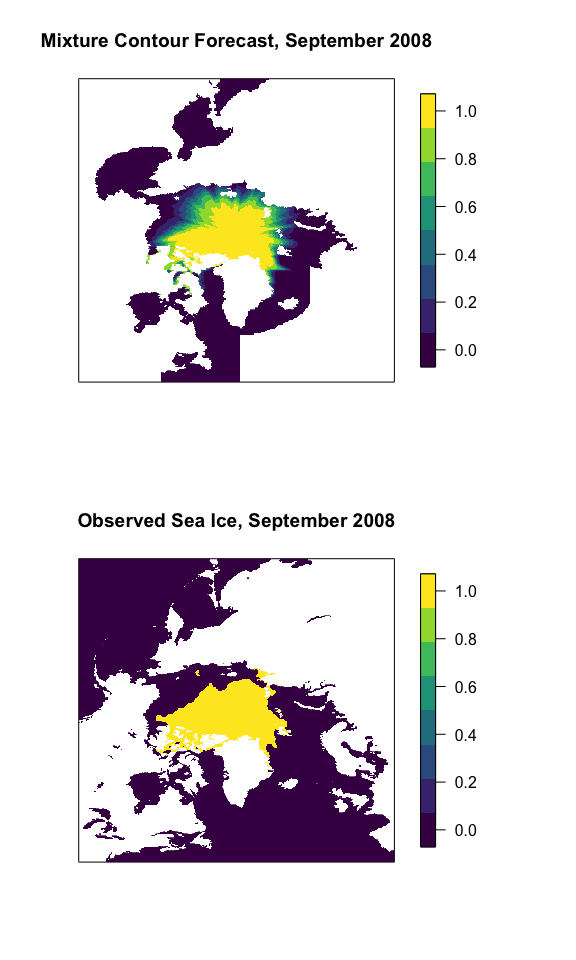# Mixture Contour Forecasting

## Introduction

This vignette introduces how to weight two probabilistic sea ice forecasts as in Probabilistic Forecasting of the Arctic Sea Ice Edge with Contour Modeling (Director et al. 2019+). In Director et al. (2019+), two forecasts are weighted to produce an overall forecast: one from the post-processed ensemble and the other from the climatology in the last ten years. We’ll fit the forecast for September 2008 using data from September 2005-2007 at a 2.5-month lead time.

## Fitting Weights

The weights will be fit using observations and both forecast types during a 3-year training period. To get started, we’ll first load the IceCast R package.

library("IceCast")

The forecasts and observations should be arranged in an array of dimension of the number of training years x longitude x latitude. The post-processed ensemble forecast, the climatology forecast, and the observations for the training years for September 2005-2007 are stored in the package as clim_9_2005_2007, ppe_9_2005_2007, and obs_9_2005_2007. Their dimensions are all 3 x 304 x 448.

To find the weights, we input the model outputs and observations into the fit_weights function. We also need to supply a prop_area matrix, which is provided in the IceCast package for the Seas of the Arctic on a Polar Stereographic grid. This is just a matrix of dimension longitude by latitude that sums to 1. Each element gives the proportion of the total area contained in that grid box.

weight <- fit_weights(mod1 = clim_9_2005_2007, mod2 = ppe_9_2005_2007,
obs = obs_9_2005_2007, prop_area = prop_area) 

## Making a Forecast

To issue a MCF forecast for 2008, we use the weight computed in the previous step and matrices of dimension longitude x latitude that give the post-processed ensemble and climatology predictions. For September 2008, these values are stored in the package as ppe_9_2008 and clim_9_2008. We create the forecast as follows

MCF_9_2008 <- wght_mod(w = weight, mod1 = clim_9_2008, mod2 = ppe_9_2008)

Finally, we’ll plot the resulting forecast and the observation for comparison. To do this, we’ll also load a couple of packages for visualization:

library("fields")
library("viridis")
par(mfrow = c(2, 1), oma = c(0, 0, 0, 4))
image.plot(MCF_9_2008, main = "Mixture Contour Forecast, September 2008",
col = viridis(8), zlim = c(0, 1), xaxt = "n", yaxt = "n")
image.plot(obs_9_2008, main = "Observed Sea Ice, September 2008",
col = viridis(8), zlim = c(0, 1), xaxt = "n", yaxt = "n")